Warning: Illegal string offset 'html' in /home/hsn/public_html/forum/cache/skin_cache/cacheid_1/skin_topic.php on line 909

differential questions ... - HSN forum# differential questions ...

12 replies to this topic

### #1cK_hoangan

• Gender:Not Telling

Posted 27 November 2007 - 06:18 PM

Pls, help me these 2 questions

1. y = (lnx)^(√x) , dy/dx ?

2. y= sin2 (x) / (1+cotg x) + cos2 (x) / (1+tan x) , dy/dx = ?

### #2George

Child Prodigy

••• 720 posts
• Location:West Lothian
• Gender:Male

Posted 27 November 2007 - 10:08 PM

For the first, you should use logarithmic differentiation: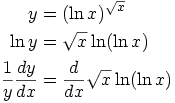Then the only difficulty is doing that derivative on the RHS - it involves the product rule, and plenty of the chain ruleAs for the second one, I'm not sure how much to give awayI used Maple (computer software) to evaluate it, and the simple answer it gave suggested to me that there's a nice way to simplify the expression before differentiating.

The first step I took was to multiply everything in the 1st fraction by, giving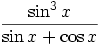, and doing a similar thing with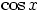on the 2nd fraction, you should get this: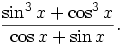After a little bit of thought, I ended up multiplying top and bottom by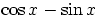. After that, find afactor (plus other stuff) in the numerator, and you should get some cancellation.

In the end,and so. ObviouslyIf you want more help with getting to, just post again### #3cK_hoangan

• Gender:Not Telling

Posted 28 November 2007 - 12:55 PM

I'm really impressed. How old r u ?

By the way, actually, when u reach to sin3 (x) + cos3 (x) / ( cos (x) + sin (x)) we do have a shorter way to simplify :

Remember : sin3 (x) + cos3 (x) = (sin (x) + cos (x)) (sin2 (x) - sinxcosx + cos2 (x)) A^3 + B^3=(A+B)(A^2-AB+B^2)
= (sin (x) + cos (x)) (1 - sinxcosx) sin2 (x) + cos2 (x) = 1
= (sin (x) + cos (x)) (1 - 1/2 sin (2x)) sin (2x) = 2 sinx cosx

hence ((sin (x) + cos (x)) will be simplified to end up with the form y = 1-1/2 sin (2x). YEAH ! You're right !!!

P/s: how could u type power and fraction when posting ? I'm a newcomer ...

### #4cK_hoangan

• Gender:Not Telling

Posted 28 November 2007 - 01:00 PM

what u got for the first question? I mean the final result ... I got it but it's so messy, a loads ...

### #5George

Child Prodigy

••• 720 posts
• Location:West Lothian
• Gender:Male

Posted 28 November 2007 - 02:02 PM

I'm really impressed. How old r u ?

Thanks, I supposeI'm 21; currently in the final year of a maths degree at Edinburgh University. How about you? These questions seem to go a bit beyond the standard Advanced Higher levelBy the way, actually, when u reach to sin3 (x) + cos3 (x) / ( cos (x) + sin (x)) we do have a shorter way to simplify :

That's much neaterFor the first question, the answer I got was:I don't think there's anything that can be done to make that look nicer!

To get the maths looking neater, you can use LaTeX code (there's an overview in this thread, with some example code).

### #6iain3915

• Gender:Male

Posted 28 November 2007 - 05:13 PM

I think for the first question to be more similar to AH maths questions it is more likely to read

y=ln(x)^(x^0.5) i.e where only the x is to power 0.5, not lnx

In which case you could have y=x^(0.5)lnx which would only involve product rule and would be at a good AH level.

### #7cK_hoangan

• Gender:Not Telling

Posted 28 November 2007 - 05:46 PM

For iain :

I got your point. But the question is really y=(lnx)^(x^0,5) which George's done very well.

but u's right, if the question's in the form y = ln (x^(x^0.5)), it's really similar to AH maths.

### #8cK_hoangan

• Gender:Not Telling

Posted 28 November 2007 - 06:00 PM

To Geogre:

I'm Vietnamese. I've just been in UK for a couple of months: exchanged student ...

My English's terrible. First few days coming here, I couldn't hear anythings, neither speaking ...

But I'm learning ... so far...

I'm 17. I attempt to go to uni in UK. With 5 subjects I'm taking now, 2 AH and 3 H I'm quite confident.

I'm really keen on Maths, so interested in me. This Friday I'm going to sit in BMO ... Do you know any website or resource I can search for Modulo. I need further about it but couldn't find out any books mention about it.

### #9George

Child Prodigy

••• 720 posts
• Location:West Lothian
• Gender:Male

Posted 28 November 2007 - 07:00 PM

Your English isn't too bad - it's certainly better than my Vietnamese!For "modulo", is this Wikipedia page the sort of thing you mean? e.g. "a is congruent to b modulo n".

I'm afraid I don't know anything about the BMO, but there may be other members here who do. Good luck, anyway!

### #10cK_hoangan

• Gender:Not Telling

Posted 28 November 2007 - 07:25 PM

Wikipedia isn't an ideal one. There's only the definition of it. What I need further about its properties, how it works and applies to solve problems.

BMO stands for British Mathematics Olympiad. I suppose you heard about it.

I have plenty of question in Maths but they're quite beyond the AH level. I wonder is it OK if I post it here...

### #11George

Child Prodigy

••• 720 posts
• Location:West Lothian
• Gender:Male

Posted 28 November 2007 - 07:56 PM

Modular arithmetic is a strange topic, as it never seems to be taught. You just tend to pick it up, bit by bit.

If you can get hold of either of the references from the Wikipedia article, you might find those more helpful:

QUOTE
Tom M. Apostol, Introduction to Analytic Number Theory, (1976) Springer-Verlag, New York. See in particular chapters 5 and 6 for a review of basic modular arithmetic. ISBN 0-387-90163-9
Thomas H. Cormen, Charles E. Leiserson, Ronald L. Rivest, and Clifford Stein. Introduction to Algorithms, Second Edition. MIT Press and McGraw-Hill, 2001. ISBN 0-262-03293-7. Section 31.3: Modular arithmetic, pp.862–868.

You can certainly ask any questions here - but I don't guarantee that I'll be able to help!Top of the Class

•• Members
•• 390 posts
• Location:Cambridge
• Interests:Muzak.
• Gender:Male

Posted 28 November 2007 - 08:35 PM

I done a bit of Olympiad maths when I was at school. My advice would be not to get too hung up on it, it gets very difficult very fast and is pretty much irrelevant to university applications.

As for learning modulo arithmetic, have a look at the Summer School lectures here - http://www.maths.ox.ac.uk/prospective-stud...te/sutton-trust. It's a very rough introduction to modular arithmetic, but it done for me last year. As George said, it's the sort of thing you get the arithmetic feel for rather than learn explicitly.

Some things I would recommend learning before going into BMO are:
Inequalities - arithmetic mean/geometric mean inequality, Triangle inequality (or inequalities), maybe the Cauchy-Schwarz inequality too but unlikely
Geometry - make sure you're confident with basic circle theorems, cyclic quadrilaterals and stuff like the cosine rule and the extended law of sines. Some people would recommend learning Ceva's theorem, Menelaus' theorem, etc., but if you use these in BMO1 you are definately over-complicating things in my opinion.
Combinatronics - all you'll need to know is familiarity with permutations and binomial coefficients. The difficulty will be in breaking the problem into manageable parts.
Modular arithmetic - Fermat's little theorem could potentially be useful for some prime factorisation, but it won't be the backbone for any of your solutions.

I don't have resources for all of these I'm afraid. Hopefully you can get the general ideas from wikipedia.
HMFC - Founded 1874, beefing the Cabbage since 1875

### #13cK_hoangan

• Gender:Not Telling

Posted 28 November 2007 - 09:39 PM

Thank you. I'm really grateful.

I'm in exciting mood and a wee bit apprehensive state. Somehow, I'm quite confident of my Maths knowledge, it's due to our 'crazy' curriculum about Maths in my country e.g. We have to remember over 100 rules and theorems of Maths such as A^3 + B^3 = ... ; cos2x=cos2 (x) - sin2 (x)=2cox2 (x)-1 ; ...etc and etc ...

All my trouble now is about language. I haven't got enough "maths terms" to use in the BMO, therefore I'm worry about my explanation when solving problems.

I did look up of some past paper of BMO. It's required a lot of knowledge in Arithmetic which is so ironically my weakness ...

Anyway, let see wat happen ...

Thank you once again.

#### 1 user(s) are reading this topic

0 members, 1 guests, 0 anonymous users